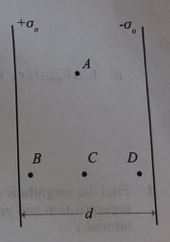# Problem: Two very large sheets of charge are separated by a distance d. One sheet +o, -O has a surface charge density +o; the other, a surface charge density- A small region near the center of the sheets is shown. 1. Draw arrows on the diagram to indicate the direction of the electric field at points A, B, C, and D. 2. Compare the magnitudes of the electric fields at points A, B, C and D. Explain.

###### FREE Expert Solution

Electric field:

$\overline{){\mathbf{E}}{\mathbf{=}}{\mathbf{-}}\frac{\mathbf{∆}\mathbf{V}}{\mathbf{∆}\mathbf{x}}}$

1.

Electric field lines are directed from high potential to low potential.

The high potential surface is positively charged while the low potential surface is negatively charged.

90% (264 ratings)###### Problem Details

Two very large sheets of charge are separated by a distance d. One sheet +o, -O has a surface charge density +o; the other, a surface charge density- A small region near the center of the sheets is shown.

1. Draw arrows on the diagram to indicate the direction of the electric field at points A, B, C, and D.

2. Compare the magnitudes of the electric fields at points A, B, C and D. Explain.